Basic Geometry : How to find the perimeter of a 45/45/90 right isosceles triangle

Example Questions

1 2 3 5 Next →

Example Question #1141 : Basic Geometry

A right isosceles triangle is stacked on top of a square as shown in the figure. Find the perimeter of the compound shape.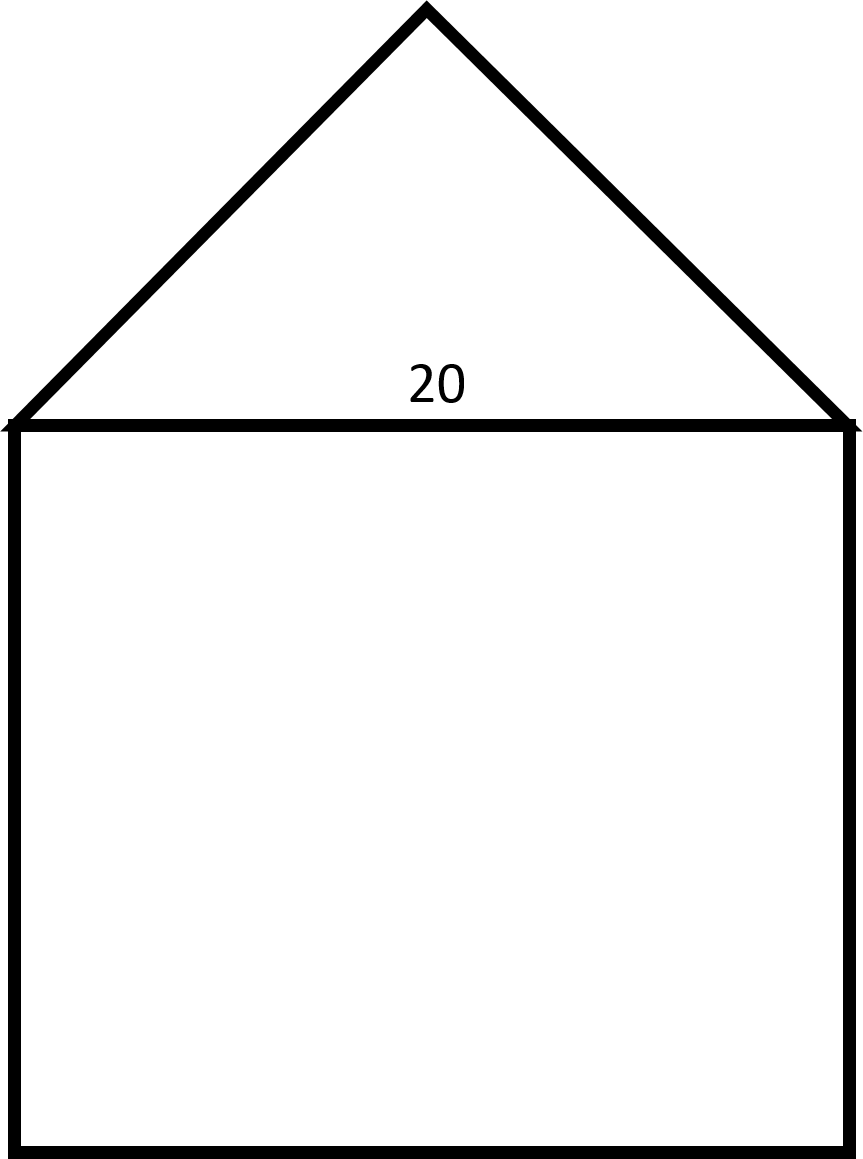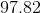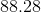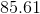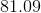Explanation: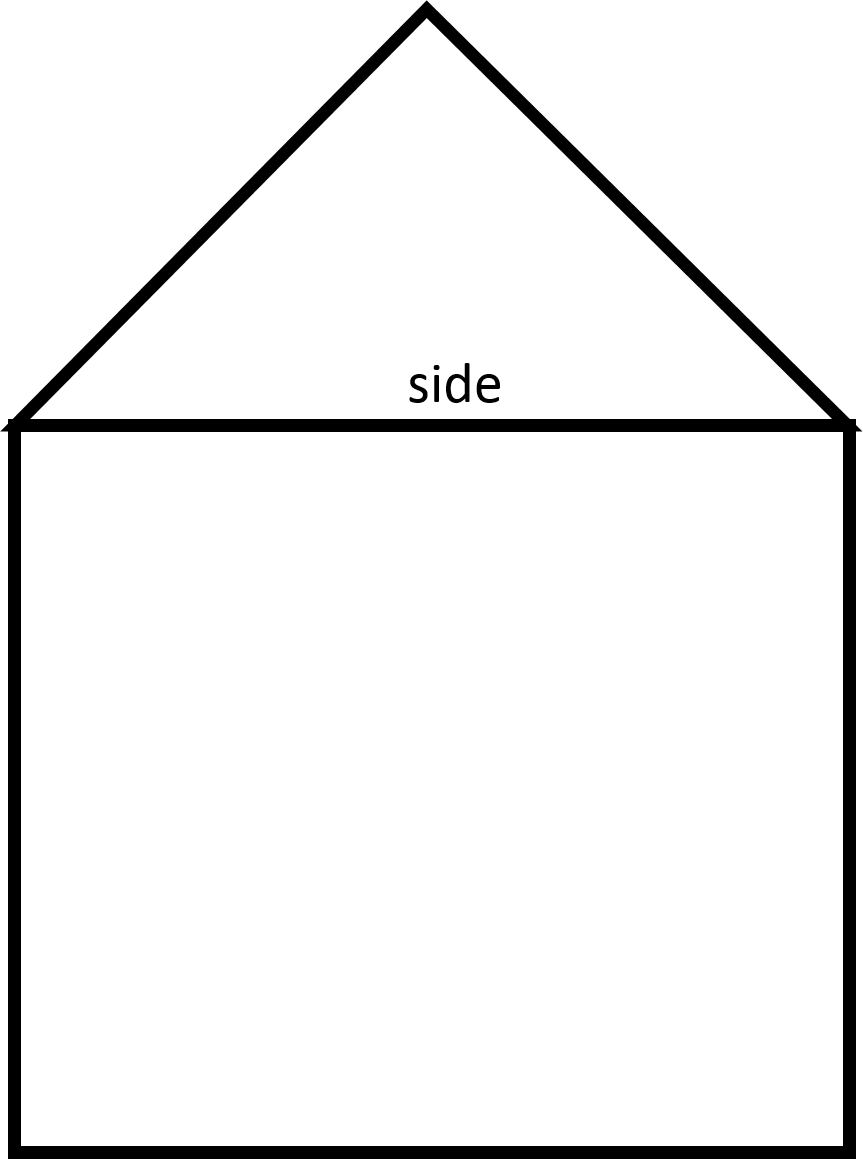Notice that the hypotenuse of the right isosceles triangle is also the length of a side of the square.

First, we will need to find the length of the legs of the triangle by using the Pythagorean theorem.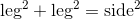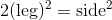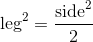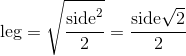Substitute in the given length of the hypotenuse to find the length of the leg of the triangle.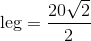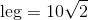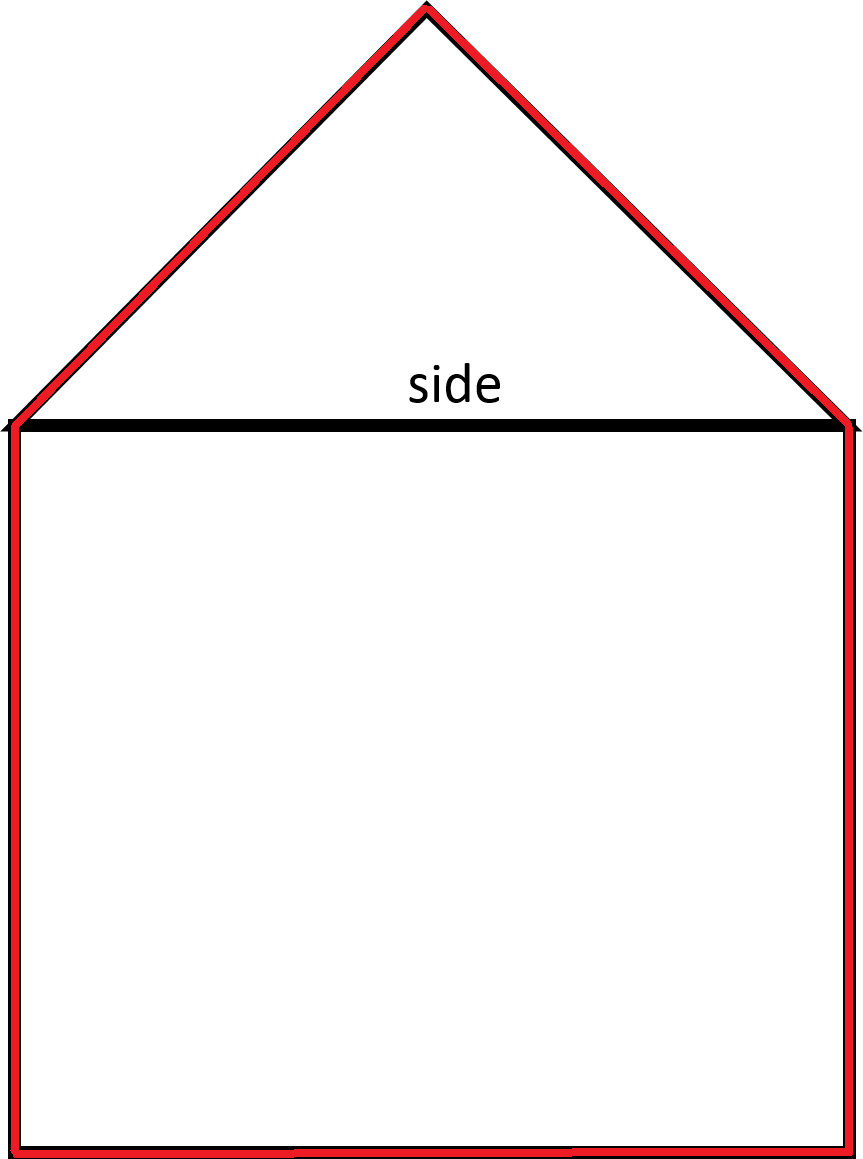In order to find the perimeter, add up the lengths outlined in red. The perimeter includes the two legs of the triangle and three sides of the square.

Therefore: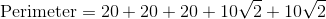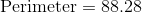Example Question #1141 : Basic Geometry

A right isosceles triangle is stacked on top of a square as shown in the figure. Find the perimeter of the compound shape.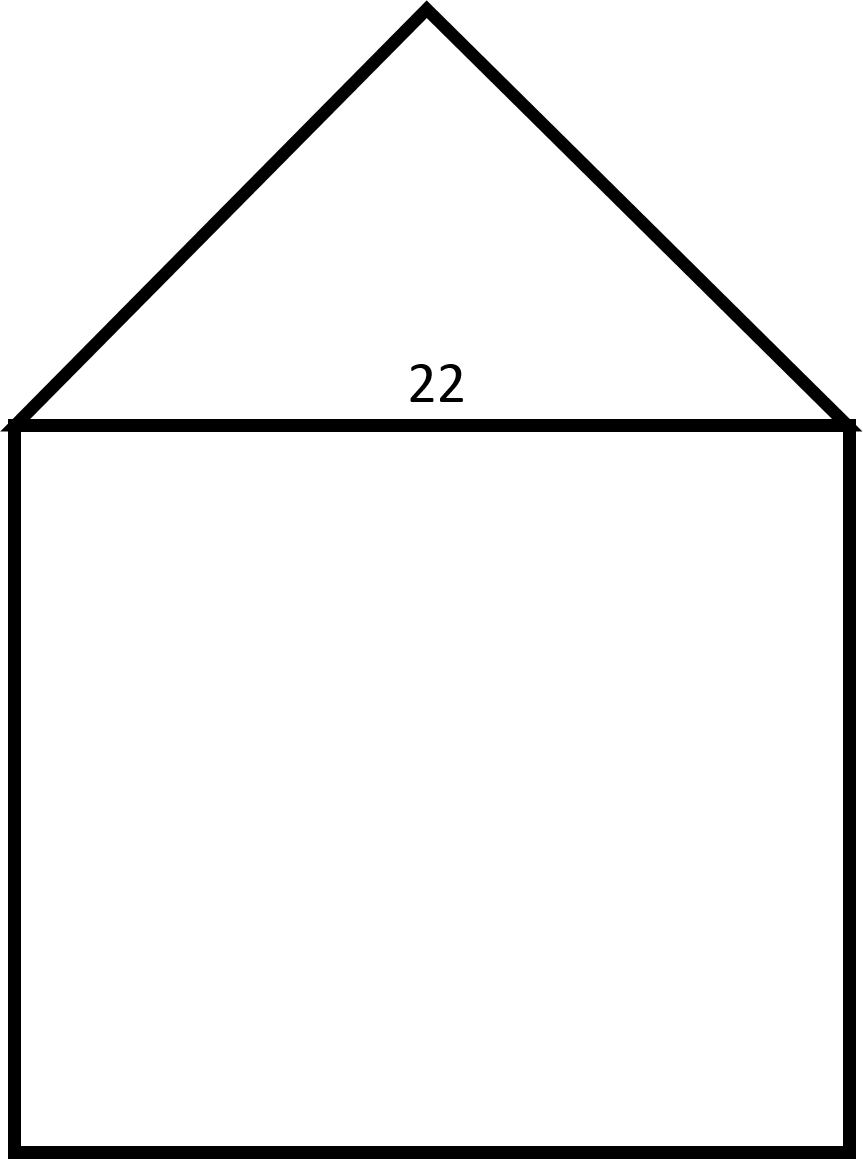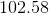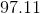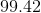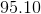Explanation:Notice that the hypotenuse of the right isosceles triangle is also the length of a side of the square.

First, we will need to find the length of the legs of the triangle by using the Pythagorean theorem.Substitute in the given length of the hypotenuse to find the length of the leg of the triangle.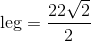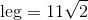In order to find the perimeter, add up the lengths outlined in red. The perimeter includes the two legs of the triangle and three sides of the square.

Therefore: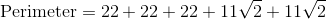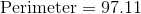Example Question #1143 : Basic Geometry

A right isosceles triangle is stacked on top of a square as shown in the figure. Find the perimeter of the compound shape.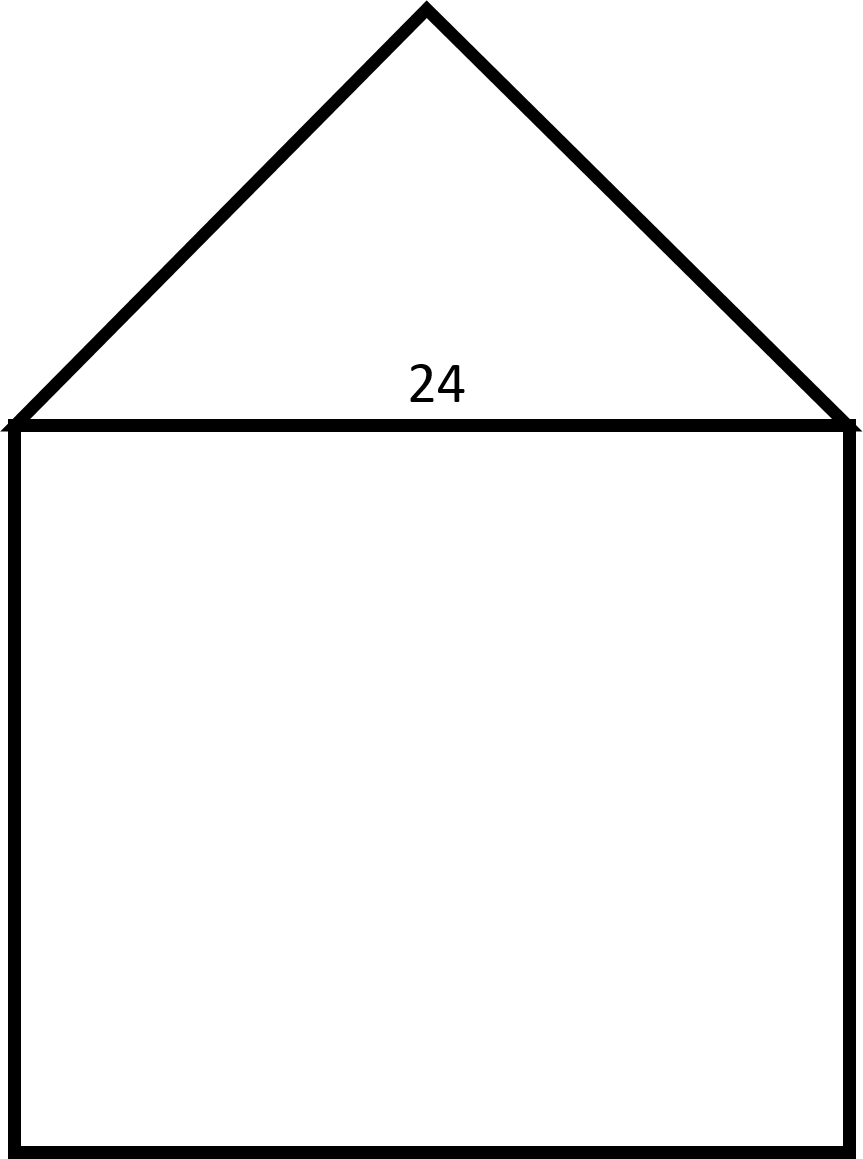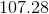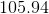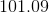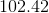Explanation:Notice that the hypotenuse of the right isosceles triangle is also the length of a side of the square.

First, we will need to find the length of the legs of the triangle by using the Pythagorean theorem.Substitute in the given length of the hypotenuse to find the length of the leg of the triangle.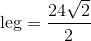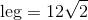In order to find the perimeter, add up the lengths outlined in red. The perimeter includes the two legs of the triangle and three sides of the square.

Therefore: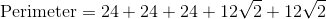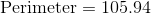1 2 3 5 Next →